# Understanding Neural Networks

Source: Deep Learning on Medium

How human biology influenced the development of AI.

Intelligence is a gift from God to mankind which helped us conquer the world and reign over every other animal on earth. This intelligence is an intangible attribute that humans benefit from, we don’t yet fully comprehend what makes it tick and how it is embedded to our brains.

All we know about the brain is that it runs on a juicy mix of blood and grey matter.😂

The human brain has long been called one of the most complicated things in science and the universe. Given its complexity, it has proven very difficult to unravel its inner workings in the past but current research is beginning to reveal its secrets.

In order to develop the field of Artificial Intelligence researchers and scientists needed to look for inspiration of an already perfect system, the human brain — this is the most fascinating organ we possess, it gives us the ability to create, innovate and a long list of things no other being on earth can do.

According to ScienceFirst, one of the top 10 facts about the brain is that there are 100 billion neurons present in it and to add to this another website called Human Memory says that every neuron can form thousands of links with other neurons in this way, giving a typical brain well over 100 trillion links (up to 1,000 trillion, by some estimates).

Please stop for a second, think about these numbers and you will see the tremendous unused power our brains have.

Imagine this, whenever you have a new experience good or bad new brain links between neurons are formed to store and represent that knowledge, your brain creates a mapping between the actions and the outcomes.

Let say, for example, you are boiling water for the first time and you touch the pot while it is still hot you will get burnt, the entire experience is recorded in a new connection and it is all done automatically, the next time you see boiling water you will remember that you will have to either wait till the pot cools down a bit or find a new way of holding the pot without being burnt, this features of the brain are the ones that inspired the creation of Artificial Neural Networks and Deep learning.

### Neural networks(NN)

(Disclaimer: I don’t want to bore you with the math behind, all I want is for you to understand the algorithm and with time we will dive deeper in to the maths.)

Neural networks(NN) are set layers of highly interconnected processing elements (neurons) that make a series of transformations on the data to generate its own understanding of it(what we commonly call features). Modelled after the human brain, NN has the goal of having machines mimic how the brain works.

The universal approximation theorem in a much simpler form states that simple neural networks can represent a wide variety of interesting functions, meaning it can solve any given problem with close accuracy when given an appropriate number of layers and neurons.

### Understanding the representation

A neural network is composed of 3 types of layers:

• Input layer — It is used to pass in our input(an image, text or any suitable type of data for NN).
• Hidden Layer — This is the layers in between the input and output. These layers are responsible for learning the mapping between input and output. (i.e. in the dog and cat gif above, the hidden layers are the ones responsible to learn that the dog picture is linked to the name dog, and it does this through a series of matrix multiplications and mathematical transformations to learn these mappings).
• Output Layer — This layer is responsible for giving us the output of the NN given our inputs.

### The engine of Neural Networks: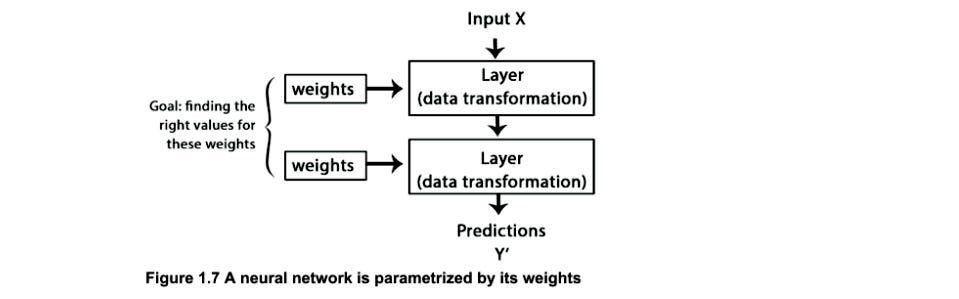All rights reserved to Deep learning with python by François Chollet

Here we will understand why I said before that NN layers transform the input data through a series of mathematical and matrix operations to learn a mapping between input and output:

output = relu( (W⋅ Input) + b)

In the expression above, W and b are tensors (multidimensional matrices) that are attributes of the layer. They are commonly called weights or trainable parameters of the layer(the kernel and bias respectively).

In the expression we do a matrix multiplication between input and weights, add the result to the bias and ReLU(Rectified Linear Unit) basically replaces all negative values with zero, that’s it, you don’t a PhD or mathematician to understand that.

These weights contain the information learned by the network from exposure to training data, in other words, the weights contain all the knowledge.

These weight matrices are filled with small random values (called random initialization), we do this because initializing all values with zero doesn’t work with Neural Networks because when we update the values, all will update to the same value repeatedly, this is not good because our network won’t be able to generalize its learning because it will always end up in the same place, so when we randomly initialize the weights we brake the symmetry.

Example: Let us take a deterministic algorithm that sort letters in alphabetical order, will systematically sort the list until you have an ordered result. Now, if given the same list, it will execute in exactly the same way. It will make the same moves at each step of the procedure.

After randomly initializing these parameters the next step is to gradually adjust these weights, based on the feedback signal (tells how far are your predictions from the real output). This gradual adjustment is commonly called training, is basically the part where the machine learns.

Training loop

• Take a fixed batch of training samples x and corresponding targets y.
• Run the network on x (a step called forward pass) to obtain predictions y_pred.
• Calculate the loss of the network on the batch, a measure of the distance between y_pred and y (loss function also called objective function= y_predy).
• Update all weights of the network in a way that slightly reduces the loss on this batch.

After a certain number of iterations(loops) your network will end up with a very low loss value(closer to zero) on its training data. Thus, the network has “learned” to map its inputs to correct targets. As François Chollet said in his book Deep learning with python:

“Learning means finding the set of values for the weights of all layers in a network, such that the network will correctly map example inputs to their associated targets.”

He also says,

“These are simple mechanisms that, once scaled, ends up looking like magic.”

### The first step in the right direction

For a long time, these algorithms had a huge problem which was an efficient way of training large NN. The first successful practical application of neural nets came in 1989 from Bell Labs, when Yann LeCun combined earlier ideas of Convolutional Neural Networks(CNN) and backpropagation.

CNN — is a class of deep neural networks, most commonly applied to analyzing images.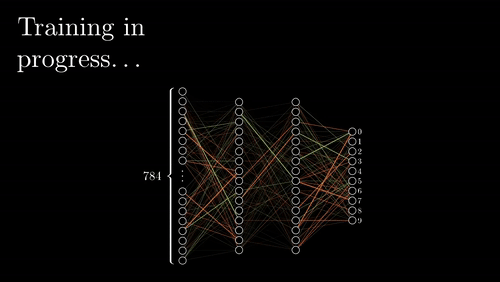Example of training an NN

BackProp — after calculation the loss function we apply chain-rule and update the weights every layer backwards to minimize the difference, get it as close to zero as possible. More on this in a future article, this is a lengthy topic.

Chain rule — is a formula for computing the derivative of the composition of two or more functions.

Listen, don’t be discouraged if you don’t understand certain concepts or words you are reading, just believe that a new link is being formed in your brain and pretty soon you will understand all of this, just keep reading and learning more.

“To succeed, one must be creative and persistent.” — John H. Johnson

### From theory to pratice

Disclaimer: (I assume you have basic programming knowledge.)

For this part, we are going to use the Python programming language and Keras High-Level Deep Learning API— both are very easy to use and understand, just follow along and try to do it yourself, it will help make those neuron links stronger.

I will release a notebook using these tools on a different dataset.

As a tradition, we have to do the hello world of deep learning which is recognizing handwritten digits.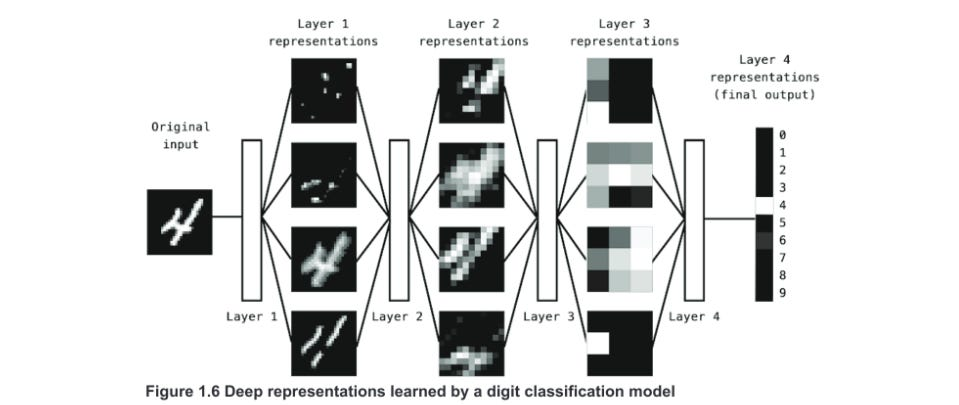All rights reserved to Deep learning with python by François Chollet

The image above describes in details what is happening inside our algorithm.

Each layer learns a different representation of the digit, as displayed in the image above one layer can learn shapes and curves and the other can learn even more abstract representations which might not make much sense to humans but it helps the algorithm classify digits better.

Steps

1. Load the MNIST dataset in Keras

2. Understand our data

The images are encoded as Numpy arrays, and the labels are an array of digits, ranging from 0 to 9. The images and labels have a one-to-one correspondence

Numpy is just a library that makes efficient mathematical and numerical operations on multidimensional arrays.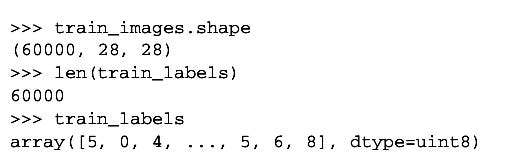All rights reserved to Deep learning with python by François Chollet

We can see that we have 60000 images in our train images variable each image with size 28×28.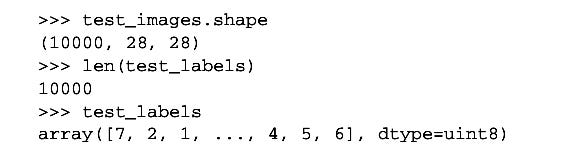All rights reserved to Deep learning with python by François Chollet

Our test data is about the same as the train set but contain fewer images about 10000 which will be kept separate and use to test the accuracy of our system.

3. Building the Neural Network

Here, our network consists of a sequence of three Dense layers, which are densely-connected (also called fully connected) neural layers. The second layer also called the hidden layer is composed of 256 neurons with ReLU(it will replace all negative values with 0) and the last layer is a10-way softmax layer, which means it will return an array of 10 probability scores (summing to 1). Each score will be the probability that the current digit image belongs to one of our 10 digit classes.

4. Defining the loss function and Optimizer(how we want to do Backprop)

5. Preprocess

We can’t just pass in data in our Neural network, first, we need to optimize the data for our Neural Network, this step is call data Preprocessing.

Basically, we’ll preprocess the data by reshaping it into the shape the network
expects and scaling it so that all values are in the [0, 1] interval.

And we transform into a matrix of 0s and 1s.

I.e. For representing the number 4 the categorical value is , this is referred to in machine learning as One-hot encoding

6. Training

Here is where we define our train loop and start training immediately.

In Keras to train a Neural Network, you use a .fit() method.

7. The final step is to evaluate how accurate is our network

This was we get to understand if the model simply memorized our training data our it actually learned something which it can use in previously unseen data.

With this model, if you follow every step you should be getting accuracy around 85–95%.

### CHALLENGE

Now you what are Neural Networks and tested it live, your challenge of the week is to apply this to a different dataset and Tweet me your GitHub profile https://twitter.com/CanumaGdt

Thank you for reading. If you have any thoughts, comments or critics please comment down below.

Follow me on twitter at Prince Canuma, so you can always be up to date with the AI field.

If you like it and relate to it, please give me a round of applause 👏👏 👏(+50) and share it with your friends.

### References

https://github.com/hktxt/bookshelf/blob/master/Computer%20Science/Deep%20Learning%20with%20Python%2C%20Fran%C3%A7ois%20Chollet.pdf